GCSE Maths Algebra Simult. Equations

Here is everything you need to know about quadratic simultaneous equations for GCSE maths (Edexcel, AQA and OCR).

You’ll learn what quadratic simultaneous equations are and how to solve them algebraically. We will also discuss their relationship to graphs and how they can be solved graphically.

Look out for the quadratic simultaneous equations worksheets and exam questions at the end.

## What are quadratic simultaneous equations?

Quadratic simultaneous equations are two or more equations that share variables that are raised to powers up to 2 e.g. x2 and y2.

Below are examples of quadratic simultaneous equations that are made up of a pair of equations; one linear equation and one equation with quadratic elements.

\begin{aligned} y&=x+3 \\ y&=x^{2}+5 x-2 \end{aligned}

\begin{aligned} y^{2}+x^{2}&=29 \\ x+7 \quad&=y \end{aligned}

\begin{aligned} 2 x^{2}-8 y^{2}&=18 \\ 3 x+4 y&=7 \end{aligned}

One key difference of quadratic simultaneous equations is that we can expect multiple answers. This is because of the way the graphs of linear and quadratic or other non-linear functions can intersect.

On the graph below we can see the straight line of the linear equation has crossed the curved parabola of the quadratic equation at two points of intersection.

This means the simultaneous equations have two valid answers.

These points of intersection are the solutions to the simultaneous equations.

E.g. Solve

\begin{aligned} x+y &=6 \\ y &=x^{2}-4 x-2 \end{aligned}

When graphed these two equations intersect at two points (− 6, 10) and (1, 3).

So the solutions to the simultaneous equations are:

$x = -6, y = 10$

and

$x = 1, y = 3$

### What are quadratic simultaneous equations?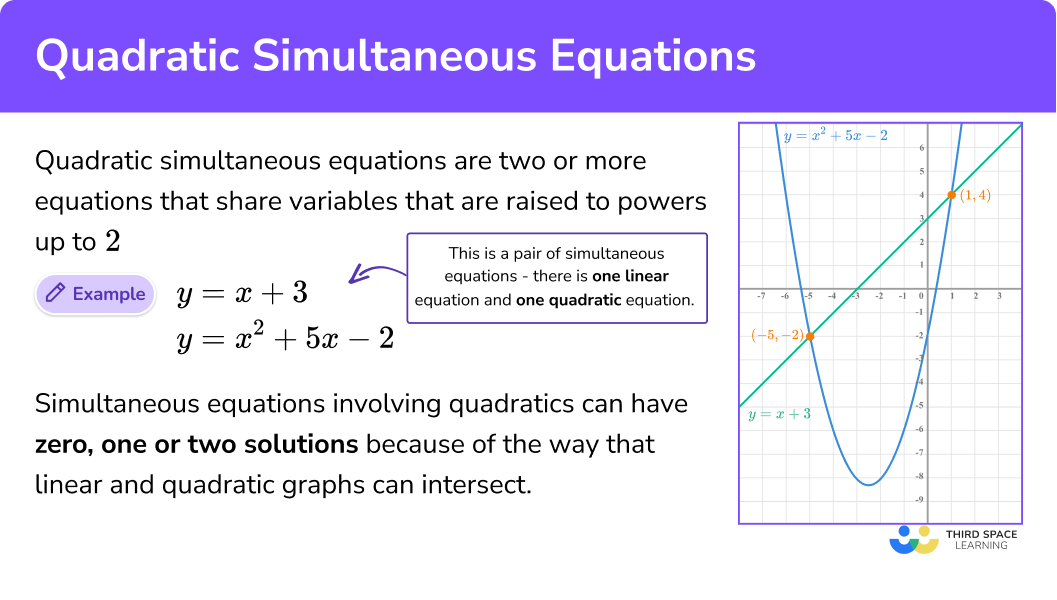Quadratic simultaneous equations is part of our series of lessons to support revision on simultaneous equations. You may find it helpful to start with the main simultaneous equations lesson for a summary of what to expect, or use the step by step guides below for further detail on individual topics. Other lessons in this series include:

## How to solve quadratic simultaneous equations

To solve a set of simultaneous equations you need to:

1. Eliminate one of the variables.
2. Find the value of one variable.
3. Find the value of the remaining variables via substitution.
4. Clearly state the final answer/s.
5. Check your answer by substituting both values into either of the original equations.

See below for example solutions to three common forms of simultaneous equations involving quadratic.

### How do you solve a set of simultaneous equations?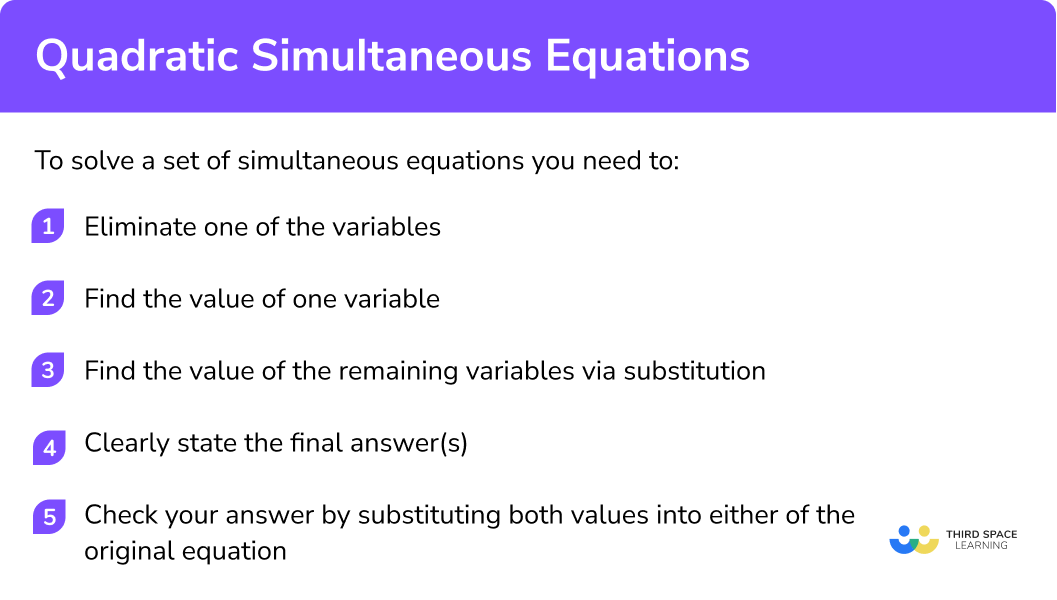### Example 1: elimination by substitution

Solve:

$\begin{array}{l} y=x+3 \\ y=x^{2}+5 x-2 \end{array}$

1. Eliminate one of the variables.

We can use the fact that y = x + 3 to substitute the value of y into the second equation.

$\begin{array}{l} y=x+3 \\ y=x^{2}+5 x-2 \end{array}$

Therefore

$x+3=x^{2}+5 x-2$

Another way to think about this is that as both equations are equal to y, they must therefore be equal to one another.

2Find the value of one variable.

As the equation is now quadratic we should make the equation = 0 before solving.

\begin{aligned} x+3 &=x^{2}+5 x-2 \\ 0 &=x^{2}+4 x-5 \\ 0 &=(x+5)(x-1)\\ x&=-5\quad or \quad x=1 \end{aligned}

NOTE: remember we are subtracting the whole of (x + 3).

NOTE: here we have solved by factorising but you could also solve by using the quadratic equation or by completing the square.

NOTE: we have found two possible values of x by using the quadratic equation.

3Find the value of the remaining variables via substitution.

As we have two values of x we can substitute both values into one of the original equations and find the two possible values of y.

Remember you can use either equation, so why not pick the easiest!

$y=x+3$

\begin{aligned} x&=-5 \\ y&=x+3 \\ y&=-5+3 \\ y&=-2 \end{aligned}

\begin{aligned} x&=1 \\ y&=x+3 \\ y&=1+3 \\ y&=4 \end{aligned}

$x=-5,y=-2$

or

$x=1,y=4$

5Check your answer by substituting both values into either of the original equations.

$y=x^{2}+5 x-2\\ x=-5, \quad y=-2$
$\begin{array}{l} -2=(-5)^{2}+5(-5)-2 \\ -2=25-25-2 \end{array}$
$y=x^{2}+5 x-2\\ x=1, \quad y=4$
$\begin{array}{l} 4=(1)^{2}+5(1)-2 \\ 4=1+5-2 \end{array}$

Both are valid so we can be confident our answer is correct.

#### Graphical representation

When graphed these two equations intersect at (− 5, − 2) and (1, 4). So the solutions to the simultaneous equations are:

$x=-5,y=-2$

or

$x=1,y=4$

### Example 2: elimination by substitution

Solve:

\begin{aligned} y^{2}+x^{2}&=29 \\ x+7&=y \end{aligned}

We can use the fact that y = x + 7 to substitute the value of y into the first equation. See below:

\begin{aligned} y^{2}+x^{2}&=29 \\ x+7&=y \end{aligned}

Therefore

$(x+7)^{2}+x^{2}=29$

As the equation is now quadratic we should make the equation = 0 before solving.

\begin{aligned} (x+7)^{2}+x^{2}&=29 \\ x^{2}+7 x+7 x+49+x^{2}&=29 \\ 2 x^{2}+14 x+49&=29 \\ 2 x^{2}+14 x+20&=0 \\ x^{2}+7 x+10&=0 \\ (x+5)(x+2)&=0 \\ x&=-5\quad or \quad x=-2 \end{aligned}

NOTE: remember (x + 7)2 means (x + 7)(x + 7).

NOTE: we can simplify by ÷ 2.

NOTE: we have found two possible values of x by using the quadratic equation.

As we have two values of x we can substitute both values into one of the original equations and find the two possible values of y.

Remember you can use either equation, so why not pick the easiest!

$x+7=y$
\begin{aligned} x&=-5\\ x+7&=y\\ -5+7&=y\\ 2&=y \end{aligned}
\begin{aligned} x&=-2\\ x+7&=y\\ -2+7&=y\\ 5&=y \end{aligned}

$x=-5,y= 2$

or

$x=-2,y=5$

$y^{2}+x^{2}=29\\ x=-5, \quad y=2$
\begin{aligned} 2^{2}+(-5)^{2}&=29 \\ 4+29&=29 \end{aligned}
$y^{2}+x^{2}=29\\ x=-2, \quad y=5$
\begin{aligned} 5^{2}+(-2)^{2}&=29 \\ 25+4&=29 \end{aligned}

Both are valid so we can be confident our answer is correct.

When graphed these two equations intersect at (− 2, 5) and (− 5, 2). So the solutions to the simultaneous equations are:

$x=-5,y= 2$

or

$x=-2,y=5$

Notice that the graph of y2 + x2 = 29 is a circle!

### Example 3: elimination by substitution with changing the subject of the formula

Solve:

\begin{aligned} 2 x^{2}-8 y^{2} &=18 \\ 3x+4y &=7 \end{aligned}

Firstly we need to make y the subject of the formula in the second equation.

\begin{aligned} 3 x+4 y &=7 \\ 4 y &=7-3 x \\ y &=\frac{7-3 x}{4} \end{aligned}

We can use the fact that

$y=\frac{7-3 x}{4}$

to substitute the value of y into the first equation. See below:

$y=\frac{7-3 x}{4}\\ 2x^{2}-8 y^{2}=18$

Therefore

$2 x^{2}-8(\frac{7-3 x}{4})^{2}=18$

As the equation is now quadratic we should make the equation = 0 before solving.

\begin{aligned} 2x^{2}-8\left(\frac{7-3 x}{4}\right)^{2} \quad&=18 \\ 2x^{2}-8\left(\frac{7-3 x}{4}\right)\left(\frac{7-3 x}{4}\right)&=18 \\ x^{2}-8\left(\frac{49-21 x-21 x+9 x^{2}}{16}\right)&=18\\ 2x^{2}-8\left(\frac{49-42 x+9 x^{2}}{16}\right)&=18 \\ 4x^{2}-\left(49-42 x+9 x^{2}\right)&=36 \\ 4x^{2}-49+42 x-9 x^{2}&=36 \\ -49+42 x-5 x^{2}&=36 \\ 42x-5x^{2} &=85\\ -5x^{2} &=85-42x\\ 0 &=5 x^{2}-42 x+85 \end{aligned}

NOTE: multiply each term by 2.

NOTE: be careful of the negatives.

NOTE: equate to 0.

This equations appears harder to factorise so we can also use the quadratic formula.

\begin{aligned} 0&=5 x^{2}-42x+85 \\ a&=5, b=-42, c=85 \\ x&=-b \pm \frac{\sqrt{b^{2}-4 a c}}{2 a} \\ x&=-(-42) \pm \frac{\sqrt{(-42)^{2}-4(5)(85)}}{2(5)} \\ x&=3.4, \qquad x=5 \end{aligned}

Notice that the solutions can be decimals as well as integers.

As we have two values of x we can substitute both values into one of the original equations and find the two possible values of y.

Remember you can use either equation, so why not pick the easiest!

\begin{aligned} x&=3.4\\ 3x+4y&=7\\ 3(3.4)+4y&=7\\ 10.2+4y&=7\\ 4y&=-3.2\\ y&=-0.8 \end{aligned}

\begin{aligned} x&=5\\ 3x+4y&=7\\ 3(5)+4y&=7\\ 15+4y&=7\\ 4y&=-8\\ y&=-2 \end{aligned}

$x= 3.4,y=-0.8$

or

$x=5,y=-2$

$2x^{2}-8y^{2}=18\\ x=3.4, \quad y=-0.8$
\begin{aligned} 2(3.4)^{2}-8(-0.8)^{2}&=18 \\ 23.12-5.12&=18 \end{aligned}
$2x^{2}-8y^{2}=18\\ x=5, \quad y=-2$
\begin{aligned} 2(5)^{2}-8(-2)^{2}&=18 \\ 50-32&=18 \end{aligned}

Both are valid so we can be confident our answer is correct.

When graphed these two equations intersect at (3.4, − 0.8) and (5, − 2). So the solutions to the simultaneous equations are:

$x= 3.4,y= -0.8$

or

$x=5,y=-2$

### Common misconceptions

• Negative numbers

Making small mistakes when +, −, ✕, ÷ with negative numbers.

Remember when squaring a negative number you will get a positive.

• Missing solutions

It is easy to forget that quadratic simultaneous equations can have two pairs of solutions. We need to use substitution to find all of the possible solutions.

• Not checking the answer using substitution

Errors can quickly be spotted by substituting your solutions in the original first or second equations to check they work.

• Algebraic fractions

When using algebraic fractions to remove the denominator make sure each term is carefully multiplied.

E.g.

$2 x^{2}-\frac{49-42 x+9 x^{2}}{2}=0\\ 4 x^{2}-(49-42 x+9 x^{2})=0$

NOTE: multiply each term by 2.

### Practice quadratic simultaneous equations questions

1. Solve the Simultaneous Equation:

y=x+1
y=x^{2}+3 x-2Because we know  y=x+1 ,  we can substitute an expression for  y into the second equation, so that  x+1=x^{2}+3x-2 .

We then use our standard techniques to solve this quadratic equation. Substitute both answers for x into the first equation to find the necessary  y values.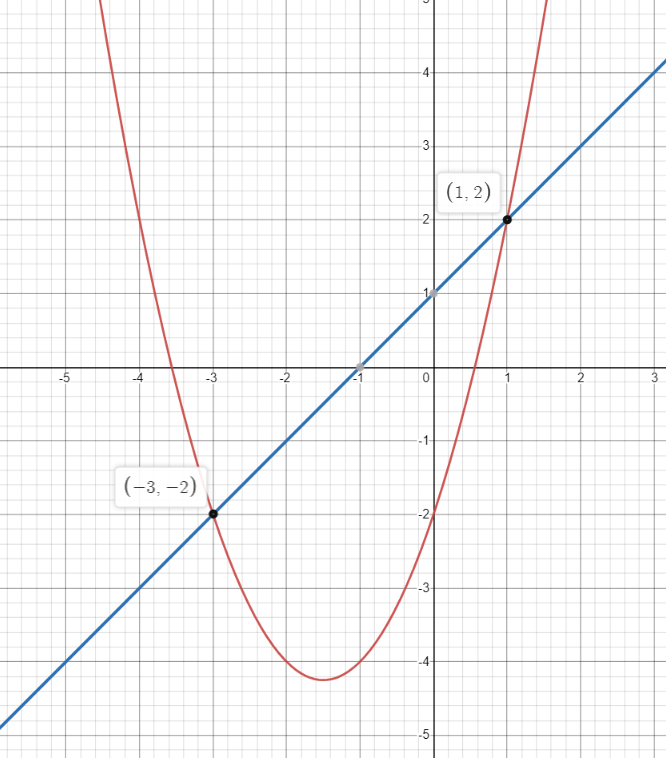2. Solve the Simultaneous Equation:

x+y =4
y=x^{2}+3 x-1Rearranging the first equation gives  y=4-x

We can substitute this into the second equation, giving  4-x=x^{2}+3 x-1

We then use our standard techniques for solving quadratic equations. Substitute both answers for x into the first equation to work out the necessary y values.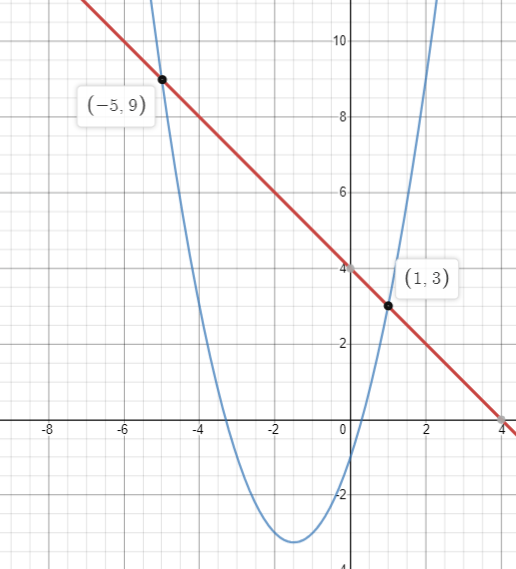3. Solve the Simultaneous Equation:

y=9 x^{2}+11 x+3
5 x-y =-2Rearranging the first equation gives  y=5x+2

We can substitute this into the second equation, giving  5x+2=9 x^{2}+11 x+3

We then use our standard techniques for solving quadratic equations. This equation has a repeated root, so there is only one pair of solutions.

There is only one pair of solutions so the graphs only intersects at one point.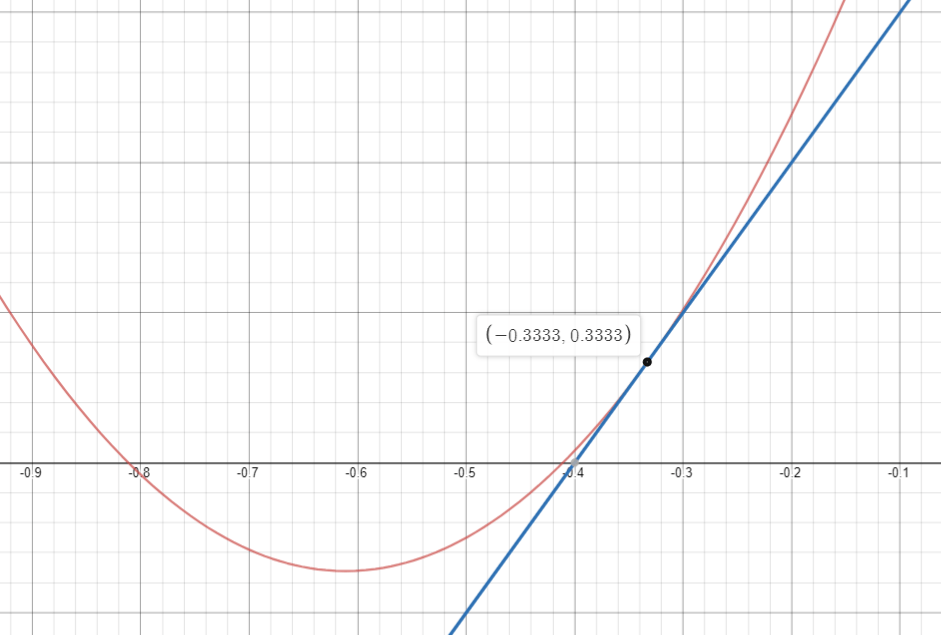4. Solve the Simultaneous Equation:

y =x-2
2 x^{2}-x y =11Substituting the first equation into the second gives  2 x^{2}-x (x-2) =11   or  x^{2}+2x-11=0 .

We then use our standard techniques for solving quadratic equations. This can be solved by completing the square, but it is probably easier to use the quadratic formula in this case.

Substitute both answers for x into the first equation to work out the necessary y values.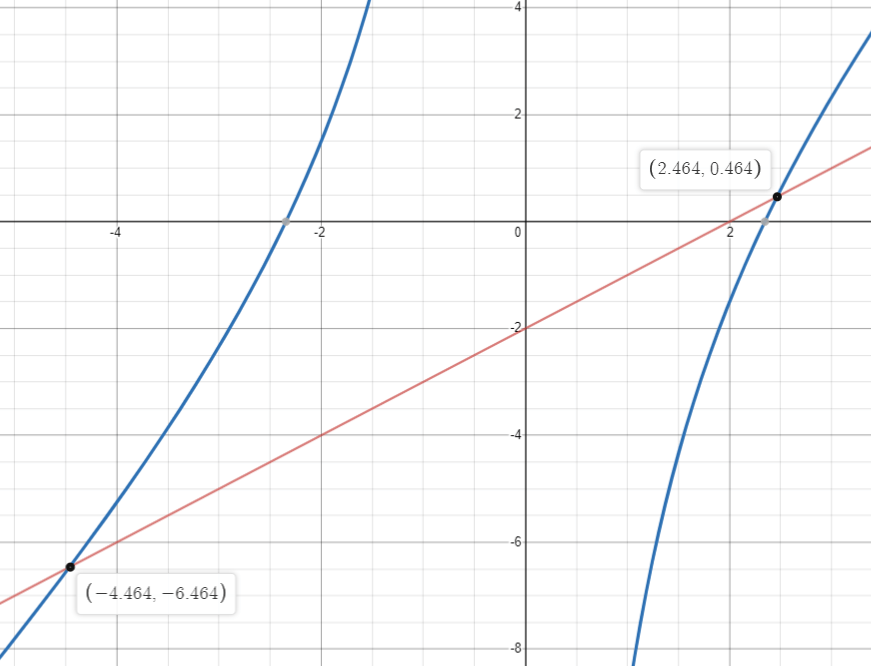### Quadratic simultaneous equations GCSE questions

1. Solve the simultaneous equations:

x y =12
y-3 x+9 =0

(7 marks)

Rearranging second equation to y = 3x − 9

oe (1)

Substitution of (3x − 9) into equation one

(1)

Correct expansion of bracket, e.g.

3 x^{2}-9 x=12

(1)

Equation = 0

(1)

Solving for x correctly ( 4 and 1 )

Correct substitution of x back into either equation

ft (1)

(2)

2. Solve the simultaneous equations:

\begin{aligned} a^{2}+b^{2}&=20 \\ b+10&=2 a \end{aligned}

(5 marks)

Clear attempt for substitution of (10 − 2a) into first equations

(1)

Correct expansion of at least 3 terms of (10 − 2a)^{2} seen

100-20 a-20 a+4 a^{2}

(1)

Simplifying to:

5 a^{2}-40 a+80 \text { or } a^{2}-8 a+16

(1)

Correct substitution of a back into either equation for b

ft (1)

a= 4 , b=-2

(1)

3. Solve algebraically the simultaneous equations. You must show your working out at each stage:

\begin{aligned} x^{2}-4 y^{2}&=9 \\ 3 x+4 y&=7 \end{aligned}

(6 marks)

A method to find an expression for y to substitute into first equations

E.g.

y=\frac{7-3 x}{4}

(1)

Correct substitution and expansion

E.g.

x^{2}-4\left(\frac{49-42 x+9 x^{2}}{16}\right)=9

or

4 x^{2}-49+42 x-9 x^{2}=36

(1)

Correct attempt to form a quadratic equation

E.g.

5 x^{2}-42 x+85=0

(1)

Correct method for solving quadratic equations

(1)

\begin{aligned} &x=\frac{17}{5}, y=-\frac{4}{5} \\ &x=5, y=-2 \end{aligned}

(2)

## Learning checklist

• Solve two simultaneous equations with two variables (where one equation is linear and the other is quadratic) algebraically

## Still stuck?

Prepare your KS4 students for maths GCSEs success with Third Space Learning. Weekly online one to one GCSE maths revision lessons delivered by expert maths tutors.

Find out more about our GCSE maths tuition programme.